# Problem 52936. Easy Sequences 39: Perfect Squares in Pascal's Triangle

Consider the 2nd, 3rd and 4th diagonals of the Pascal's Triangle, shown highlighted below: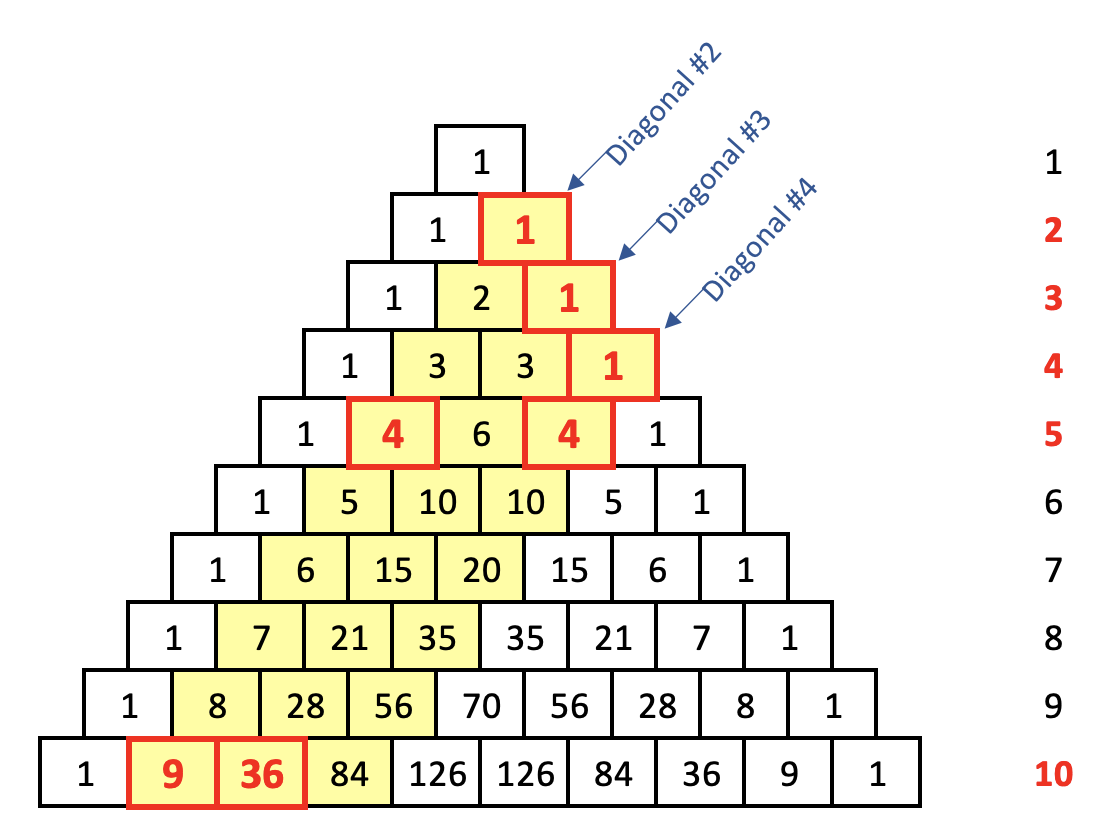We can see that on the 2nd, 3rd, 4th, 5th and 10th rows, the highlighted diagonals contains at least one perfect square elements:
• 2nd Diagonal: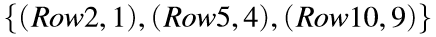• 3rd Diagonal: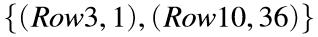• 4th Diagonal: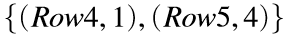Given a row limit r, write a function that outputs a set of row numbers , in which the 2nd, 3rd and 4th diagonals of the pascal's triangle contains at least one perfect square elements.
In the case above where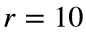, the function should return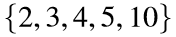.

### Solution Stats

72.73% Correct | 27.27% Incorrect
Last Solution submitted on Mar 19, 2023

### Community Treasure Hunt

Find the treasures in MATLAB Central and discover how the community can help you!

Start Hunting!